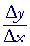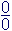An Approach

to

C A L C U L U S

2

# LIMITS

A sequence of rational numbers

Section 2:

CENTRAL TO CALCULUS is the value of the slope of a line,, but when the terms approach. Evaluating that rate of change under those vanishing conditions requires the idea of a limit. And central to the idea of a limit is the idea of a sequence of rational numbers.

## A sequence of rational numbers

We encounter such a sequence in geometry when we determine a formulafor the area of a circle.  To do that, we inscribe in the circle a regular polygon of n sides.  The area of the polygon, which we can actually calculate, will be an approximation to the area of the circle. As we increase the number of sides -- that is, if we consider a sequence of polygons:  60 sides, 61 sides, 62, 63, 64, and so on -- then the sequence of those areas becomes closer and closer to the area of the circle.  Now, the circle is never equal to a polygon. But by considering a sufficiently large number of sides, the difference between the circle and that polygon will be less than any small number we specify.  Less, say, than

0.00000000000000000000000000000001

That is the idea of a sequence approaching a limit, or a boundary, which in this example is the area of the circle.

The student surely can recognize the number that is the limit of this sequence of rational numbers.

3,  3.1,  3.14,  3.141,  3.1415,  3.14159,  3.141592, . . .

π

We speak of a sequence being infinite, which, in analogy with the sequence of natural numbers, is a brief way of saying that, because of a rule or a pattern or a procedure, there is no limit to the number of terms we coulld name.

## The limit of a variable

Consider this sequence of values of a variable x:

1.9,  1.99,  1.999,  1.9999,  1.99999, . . .

Now, no member of that sequence will every equal 2. We say, however, that those values are approaching 2 as their "limit."  Why? Because 2 is the smallest number such that no matter which term of the sequence we name, it will be less than 2.

In other words, it will be possible to name a term of that sequence such that the absolute value of the difference between that term and 2 --

|1.999999999999 −2|

-- will be less that any positive number we name, however small.  (Definition 2.1, below.)

(We write the absolute value because the terms are less than 2, and so the difference itself will be negative.)

When the values of x approach a number l as a limit, we symbolize that as  xl.  Read:  "The values of x approach l as a limit," or simply, "x approaches l."  In the example above,  x2.  "x approaches 2."

(How else would x "approach" a value, other than as a sequence of rational numbers?)

We also say that a sequence converges to a limit.  The sequence above converges to 2.

By a sequence in what follows, we mean an ordering of rational numbers according to a rule or an indicated pattern.  Here, for example, is a sequence that approaches 0:

0.1,  0.01,  0.001,  0.0001,  0.00001,  and so on.

## Left-hand and right-hand limits

Now the sequence we chose were values less than 2:

1.9,  1.99,  1.999,  1.9999,  1.99999, . . .

And so we say that x approaches 2 from the left.  We write

x2−

But we can easily construct a sequence of values of x that converges to 2 from the right; that is, a sequence of values that are more than 2.
For example,

2.2,  2.1,  2.01,  2.001,  2.0001,  2.00001, .  .  .

In this case, we write  x2+ .

But again, no matter what small number we specify, if we go far enough out in that sequence, the value of |x − 2| will be less than that small number.  And so will all subsequent differences we might name.

Again, when we say that the values of x "approach a limit," that limit -- that number -- is never a value of x.  There is always a difference between the values of x and their limit.  The limit is the boundary beyond which no member of the sequence will pass.

We summarize this in the following definition.  But first, x is not the only variable.  y is a variable.  And y will be a function of x -- f(x) -- which is also a variable.  And when we come to the definition of the derivative, Δx or h will be the variable.  In the following, then, we will use the letter v to represent any variable.

DEFINITION 2.1.  The limit of a variable.  We say that a sequence of values of a variable v approaches a number l as a limit (a number not a term in the sequence), if, beginning with a certain term vn, and for any subsequent term we might name, the absolute value of vn − l  is less than any positive number we name, however small.

When that condition is satisfied, we write  vl.

And so when the values of a variable approach a limit, there is always a difference between the limit and those values.  But that difference can be made as small as we please. That is the essence of a variable approaching a limit.

If Δx is the variable that approaches the limit 0 (as it does when we determine the derivative), then Δx is never equal to 0.

-

## The limit of a function of a variable

We have defined the limit of a variable, but what we often have is a function of a variable -- which is itself a variable.  For example,

y = f(x) = x2.

Now, a sequence of values of x, the independent variable, will cause a sequence of values of f(x), the dependent variable. The question is:  As the values of x approach a limit c, will the corresponding values of f(x) approach a limit L ?  If that is the case, then we write"The limit of f(x) as x approaches c  is L."

In fact, let us see what happens to  f(x) = x2  as x2−.  Suppose again that x assumes this sequence:

1.9,  1.99,  1.999,  1.9999,  1.99999, . . .

x2 will then become this sequence:

1.92,  1.992,  1.9992,  1.99992,  1.999992, . . .

It is easy to see that x2 approaches 22 = 4.That is, if we go far enough out in the sequence of values of x, then the differences between the x2s and 4 --

|1.92 − 4|,  |1.992 − 4|, |1.9992 − 4|, |1.99992 − 4|, |1.999992 − 4|, . . .

-- will become less than any positive number we specify, however small. The definition of the limit of a variable will be satisfied.  f(x) = x2 will approach 4 as a limit as x approaches 2.

Moreover, if x approaches 2 from the right:

2.2,  2.1,  2.01,  2.001,  2.0001,  2.00001, .  .  .

then those values cause x2 to become this sequence:

2.22,   2.12,   2.012 ,   2.0012,   2.00012,  .  .  .

That sequence also will approach 4.  Therefore, the limit of x2 as x approaches 2 both from the right and from the left is the same number. Therefore we can drop the + or − signs and simply write:To summarize:

DEFINITION 2.2.  The limit of a function of a variable.We say that a function f(x) approaches a limit L as x approaches c  if the sequence of values of x, both from the left and from the right, causes the sequence of values of f(x) to satisfy the definition of "approaches a limit": Definition 2.1.

If that is the case, then we write:"The limit of f(x) as x approaches c  is  L."

Thus for the limit of a function to exist as the independent variable approaches c , the left-hand and right-hand limits -- those numbers -- must be equal.if and only ifWhen we say, then, that a function approaches a limit, we mean that Definition 2.2 has been satisfied. The theorems on limits imply that.

The most important limit -- the limit that differential calculus is about -- is called the derivative. All the other limits studied in Calculus I are logical fun and games, never to be heard from again.

Now here is an example of a function that does not approach a limit:As x approaches 2 from the left,  f(x) approaches 1.  As x approaches 2 from the right,  f(x) approaches 3.  The left- and right-hand limits are not equal.  Therefore,  f(x) does not approach any limit as x approaches 2. Definition 2.2,

In Topic 3 we will see that f(x) is not continuous at x = 2.

Section 2:  Theorems on limits

Please make a donation to keep TheMathPage online.
Even \$1 will help.